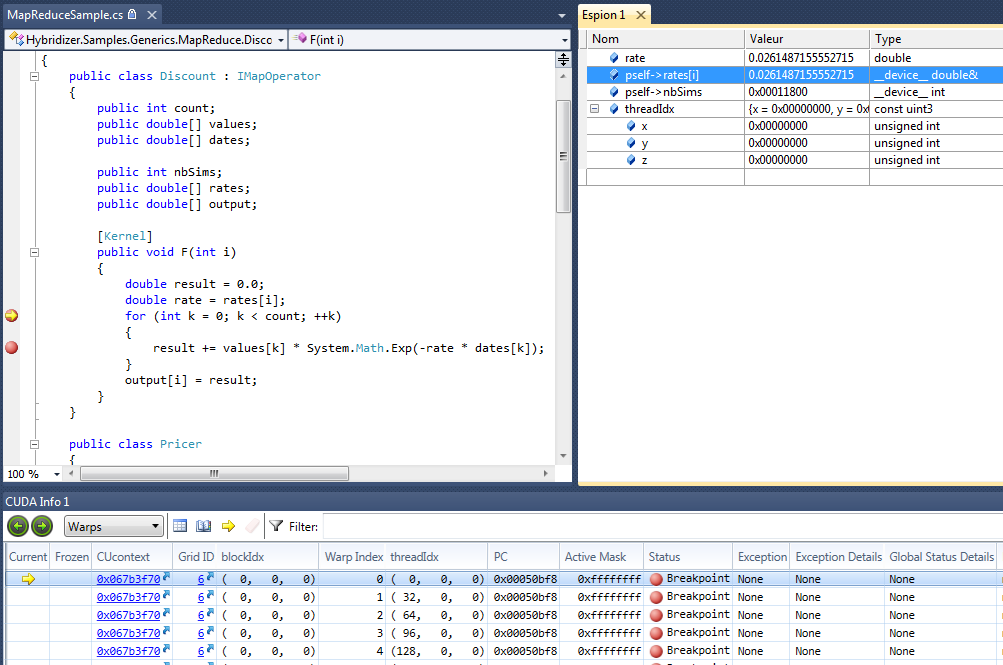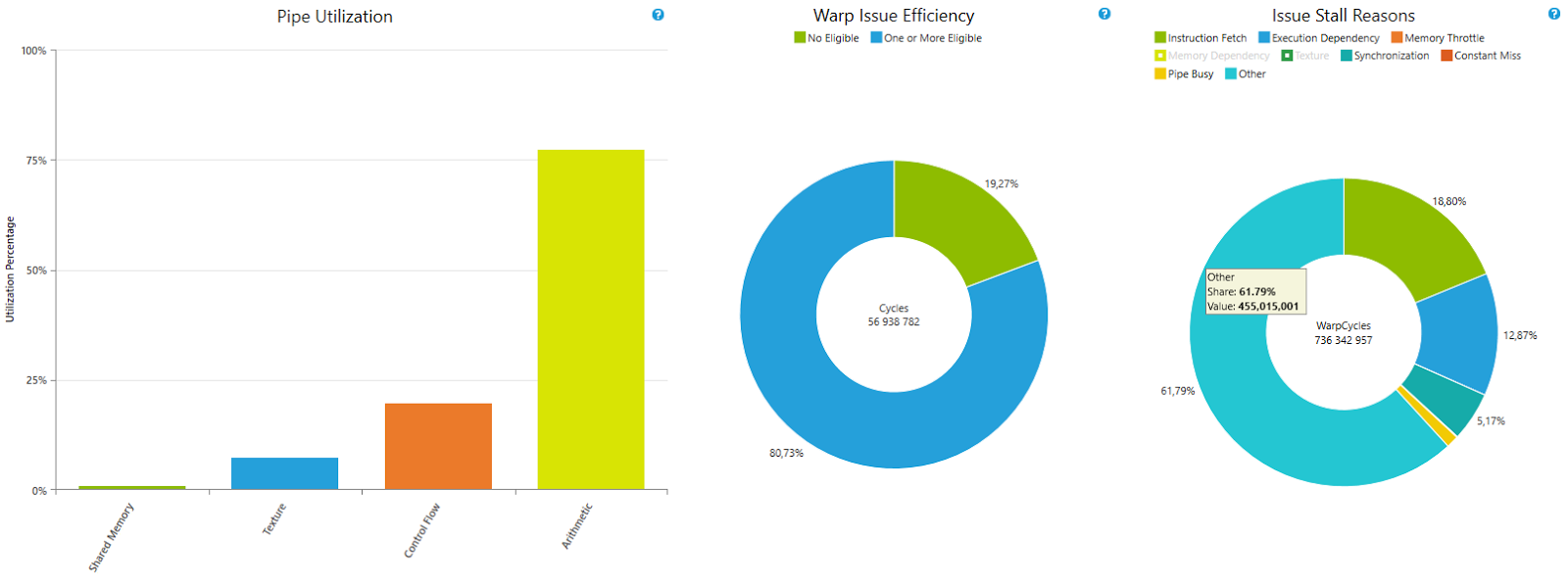# Hybridizer: High-Performance C# on GPUsHybridizer is a compiler from Altimesh that lets you program GPUs and other accelerators from C# code or .NET Assembly. Using decorated symbols to express parallelism, Hybridizer generates source code or binaries optimized for multicore CPUs and GPUs. In this blog post we illustrate the CUDA target.

Figure 1 shows the Hybridizer compilation pipeline. Using parallelization patterns such as `Parallel.For`, or by distributing parallel work explicitly as you would in CUDA, you can benefit from the compute horsepower of accelerators without learning all the details of their internal architecture. Here is a simple example using `Parallel.For` with a lambda.

```[EntryPoint]
public static void Run(double[] a, double[] b, int N)
{
Parallel.For(0, N, i => { a[i] += b[i]; });
}```

You can debug and profile this code on the GPU using NVIDIA Nsight Visual Studio Edition. Hybridizer implements advanced C# features including virtual functions and generics.

## Where to Get Hybridizer

Hybridizer comes in two versions:

While providing automated default behavior, Hybridizer gives full developer control at each phase, allowing you to reuse existing device-specific code, existing external libraries or custom handmade code snippets.

## Debugging And Profiling

When compiled with debug information, you can debug Hybridizer C# / .NET code  within Microsoft Visual Studio while running the optimized code on the target hardware. For example, a program written in C# can hit a breakpoint in the C# file within Visual Studio and you can explore local variables and object data that reside on the GPU.Figure 2: Debugging C# code running on the GPU with Hybridizer and NVIDIA Nsight Visual Studio Edition.

You can integrate Hybridizer within complex projects, even in libraries for which code is not available or is obfuscated, because Hybridizer operates on MSIL bytecode. We demonstrated this ability in our blog post about accelerating the AForge image processing library with Hybridizer without modifying the library. Operating on MSIL bytecode also enables support for a variety of languages built on top of the .Net virtual machine, such as VB.Net and F#.

All this flexibility does not come at the expense of performance loss. As our benchmark illustrates, code generated by the Hybridizer can perform as well as hand-written code. You can use performance profilers such as NVIDIA Nsight and the NVIDIA Visual Profiler to measure performance of generated binaries, with performance indicators referring to the original source code (C#, for instance).

## A Simple Example: Mandelbrot

As a first example, we demonstrate the rendering of the Mandelbrot fractal running on an NVIDIA GeForce GTX 1080 Ti GPU (Pascal architecture; Compute Capability 6.1).

### Mandelbrot C# Code

The following code snippet shows plain C#. It runs smoothly on the CPU without any performance penalty, since most code modifications are attributes (such as the `EntryPoint` attribute on the `Run` method) which have no effect at run time.

```[EntryPoint]
public static void Run(float[,] result)
{
int size = result.GetLength(0);
Parallel2D.For(0, size, 0, size, (i, j) => {
float x = fromX + i * h;
float y = fromY + j * h;
result[i, j] = IterCount(x, y);
});
}

public static float IterCount(float cx, float cy)
{
float result = 0.0F;
float x = 0.0f, y = 0.0f, xx = 0.0f, yy = 0.0f;
while (xx + yy <= 4.0f && result < maxiter) {
xx = x * x;
yy = y * y;
float xtmp = xx - yy + cx;
y = 2.0f * x * y + cy;
x = xtmp;
result++;
}
return result;
}
```

The `EntryPoint` attribute tells the Hybridizer to generate a CUDA kernel. Multi-dimensional arrays are mapped to an internal type, while `Parallel2D.For` maps to a 2D execution grid. Given a few lines of boilerplate code, we run this code on the GPU transparently.

```float[,] result = new float[N,N];
HybRunner runner = HybRunner.Cuda("Mandelbrot_CUDA.dll").SetDistrib(32, 32, 16, 16, 1, 0);
dynamic wrapper = runner.Wrap(new Program());
wrapper.Run(result);```

### Profiling

We profiled this code with the Nvidia Nsight Visual Studio Edition profiler. C# code is linked to the PTX in the CUDA source view, as Figure 3 shows.

The profiler allows the same level of investigation as with CUDA C++ code.

As for performance, this example reaches 72.5% of peak compute FLOP/s. This is 83% of the same code, handwritten in CUDA C++.Figure 4: Profiler output showing the GPU utilization and execution efficiency of the Mandelbrot code on the GPU. It achieves nearly as good efficiency as handwritten CUDA C++ code.

Obtaining better performance from C# code is possible using the extended control that Hybridizer provides. As the following code shows, the syntax is very similar to CUDA C++.

```[EntryPoint]
public static void Run(float[] result)
{
for (int i = threadIdx.y + blockIdx.y * blockDim.y; i < N; i += blockDim.y * gridDim.y)
{
for (int j = threadIdx.x + blockIdx.x * blockDim.x; j < N; j += blockDim.x * gridDim.x)
{
float x = fromX + i * h;
float y = fromY + j * h;
result[i * N + j] = IterCount(x, y);
}
}
}```

In this case, generated code and handwritten CUDA C++ code perform identically and reach 87% of peak FLOP/s, as Figure 5 shows.

## Generics And Virtual Functions

Hybridizer supports generics and virtual function calls in device functions. These fundamental concepts of modern programming languages facilitate code modularity and increase expressivity. However, type resolution in C# is done at run time, which introduces some performance penalty. .NET generics can achieve higher performance while maintaining flexibility: Hybridizer maps generics to C++ templates, which are resolved at compile time, allowing function inlining and interprocedural optimizations. On the other hand, virtual function calls are mapped to a virtual function table in which instance methods are registered.

Template instantiation hints are given to the Hybridizer by two attributes, `HybridTemplateConcept` and `HybridRegisterTemplate` (which triggers the actual template instantiation in device code). As an example, let’s look at a simple stream benchmark in two versions, one using virtual function calls, and another with template mapping. The benchmark relies on a common interface `IMyArray` exposing subscript operators:

```[HybridTemplateConcept]
public interface IMyArray {

double this[int index] { get; set; }
}
```

These operators must be “Hybridized” to device functions. To do that, we put the `Kernel` attribute in the implementation class.

```public class MyArray : IMyArray {
double[] _data;

public MyArray(double[] data) {
_data = data;
}

[Kernel]
public double this[int index] {
get { return _data[index]; }
set { _data[index] = value; }
}
}```

### Virtual Function Calls

In a first version, we write a stream algorithm using the interface with no further hint to the compiler.

```public class MyAlgorithmDispatch {
IMyArray a, b;

public MyAlgorithmDispatch(IMyArray a, IMyArray b)  {
this.a = a;
this.b = b;
}

[Kernel]
IMyArray a = this.a;
IMyArray b = this.b;
for (int k = threadIdx.x + blockDim.x * blockIdx.x;
k < n;
k += blockDim.x * gridDim.x) {
a[k] += b[k];
}
}
}```

Since we call subscript operators on `a` and `b` viewed as interfaces, we have a `callvirt` in the MSIL.

```IL_002a: ldloc.3
IL_002b: ldloc.s 4
IL_002d: callvirt instance float64 Mandelbrot.IMyArray::get_Item(int32)
IL_0032: ldloc.1
IL_0033: ldloc.2
IL_0034: callvirt instance float64 Mandelbrot.IMyArray::get_Item(int32)
IL_003a: callvirt instance void Mandelbrot.IMyArray::set_Item(int32, float64)```

Inspecting the generated binary shows that Hybridizer generated a lookup in a virtual function table, as Figure 6 shows.

This version of the algorithm consumes 32 registers and achieves a bandwidth of 271 GB/s, as Figure 7 shows. On the same hardware, the `bandwidthTest` sample in the CUDA Toolkit achieves 352 GB/s.

Virtual function tables lead to more register pressure, and prevent inlining.

### Generic Calls

We wrote a second version with generics, asking Hybridizer to generate template code.

```[HybridRegisterTemplate(Specialize = typeof(MyAlgorithm))]
public class MyAlgorithm where T : IMyArray
{
T a, b;

[Kernel]
{
T a = this.a;
T b = this.b;
for (int k = threadIdx.x + blockDim.x * blockIdx.x;
k < n;
k += blockDim.x * gridDim.x)
a[k] += b[k];
}
}

public MyAlgorithm(T a, T b)
{
this.a = a;
this.b = b;
}
}
```

With the `RegisterTemplate` attribute, Hybridizer generates the appropriate template instance. Optimizer then inlines function calls as Figure 8 shows.

Performance of generic parameters is much better, achieving 339 GB/s, which is a 25% performance improvement (Figure 9), and 96% of `bandwidthTest`.